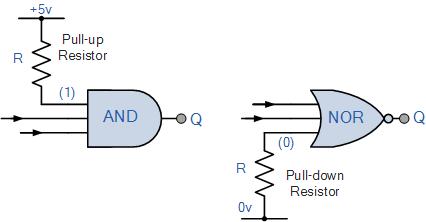# Digital Logic Gates Summary

## Digital Logic Gates Summary

In this section about Digital Logic Gates, we have seen that there are three main basic types of digital logic gate, the AND Gate, the OR Gate and the NOT Gate. We have also seen that each gate has an opposite or complementary form of itself in the form of the NAND Gate, the NOR Gate and the Buffer respectively, and that any of these individual gates can be connected together to form more complex Combinational Logic circuits.We have also seen, that in digital electronics both the NAND gate and the NOR gate can both be classed as “Universal” gates as they can be used to construct any other gate type. In fact, any combinational circuit can be constructed using only two or three input NAND or NOR gates. We also saw that NOT gates and Buffers are single input devices that can also have a Tri-state High-impedance output which can be used to control the flow of data onto a common data bus wire.

Digital Logic Gates can be made from discrete components such as Resistors, Transistors and Diodes to form RTL (resistor-transistor logic) or DTL (diode-transistor logic) circuits, but today’s modern digital 74xxx series integrated circuits are manufactured using TTL (transistor-transistor logic) based on NPN bipolar transistor technology or the much faster and low power CMOS based MOSFET transistor logic used in the 74Cxxx, 74HCxxx, 74ACxxx and the 4000 series logic chips.

The eight most “standard” individual Digital Logic Gates are summarised below along with their corresponding truth tables.

## Standard Logic Gates

### The Logic AND Gate

 Symbol Truth Table2-input AND Digital Logic Gate B A Q 0 0 0 0 1 0 1 0 0 1 1 1 Boolean Expression Q = A.B Read as A AND B gives Q

### The Logic OR Gate

 Symbol Truth TableB A Q 0 0 0 0 1 1 1 0 1 1 1 1 Boolean Expression Q = A + B Read as A OR B gives Q

## Inverting Logic Gates

### The Logic NAND Gate

 Symbol Truth TableB A Q 0 0 1 0 1 1 1 0 1 1 1 0 Boolean Expression Q = A . B Read as A AND B gives NOT Q

### The Logic NOR Gate

 Symbol Truth TableB A Q 0 0 1 0 1 0 1 0 0 1 1 0 Boolean Expression Q = A + B Read as A OR B gives NOT Q

## Exclusive Logic Gates

### The Logic Exclusive-OR Gate (Ex-OR)

 Symbol Truth TableB A Q 0 0 0 0 1 1 1 0 1 1 1 0 Boolean Expression Q = A     B Read as A OR B but not BOTH gives Q (odd)

### The Logic Exclusive-NOR Gate (Ex-NOR)

 Symbol Truth TableB A Q 0 0 1 0 1 0 1 0 0 1 1 1 Boolean Expression Q = A     B Read if A AND B the SAME gives Q (even)

## Single Input Logic Gates

### The Hex Buffer

 Symbol Truth TableA Q 0 0 1 1 Boolean Expression Q = A Read as A gives Q

### The NOT gate (Inverter)

 Symbol Truth TableA Q 0 1 1 0 Boolean Expression Q = not A or A Read as inverse of A gives Q

The operation of the above Digital Logic Gates and their Boolean expressions can be summarised into a single truth table as shown below. This truth table shows the relationship between each output of the main digital logic gates for each possible input combination.

## Digital Logic Gate Truth Table Summary

The following logic gates truth table compares the logical functions of the 2-input logic gates detailed above.

 Inputs Truth Table Outputs For Each Gate B A AND NAND OR NOR EX-OR EX-NOR 0 0 0 1 0 1 0 1 0 1 0 1 1 0 1 0 1 0 0 1 1 0 1 0 1 1 1 0 1 0 0 1
 Truth Table Output for Single-input Gates A NOT Buffer 0 1 0 1 0 1

### Pull-up and Pull-down Resistors

One final point to remember, when connecting together digital logic gates to produce logic circuits, any “unused” inputs to the gates must be connected directly to either a logic level “1” or a logic level “0” by means of a suitable “Pull-up” or “Pull-down” resistor ( for example 1kΩ resistor ) to produce a fixed logic signal. This will prevent the unused input to the gate from “floating” about and producing false switching of the gate and circuit.As well as using pull-up or pull-down resistors to prevent unused logic gates from floating about, spare inputs to gates and latches can also be connected together or connected to left-over or spare gates within a single IC package as shown.